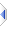# sqrt

 Definition: atom x2 = sqrt(atom x1) Description: Calculate the square root of x1. Comments: This function may be applied to an atom or sq_sqrt() to all elements of a sequence. The rules for sequence operations apply. Taking the square root of a negative number will abort your program with a run-time error message. Example: ```r = sqrt(16) -- r is 4 ``` Implementation: via :%opSqrt in builtins\VM\pTrig.e (an autoinclude) See Also: log, power